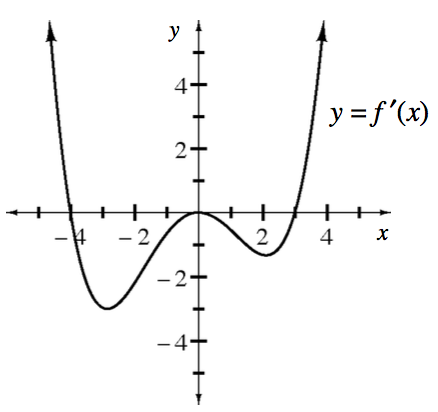### Home > APCALC > Chapter 12 > Lesson 12.1.5 > Problem12-57

12-57.

The graph at right shows the derivative of $y = f(x)$. Give approximate answers to the questions below and justify your responses.

1. For what value(s) of $x$ does $f$ attain a relative minimum?

This occurs when $f′(x)$, or the slope function, changes from negative to positive.

2. At what value(s) of $x$ does a critical point that is neither a relative maximum nor a relative minimum exist?

This occurs when $f′(x) = 0$, but does not change from negative to positive.

3. For what values of $x$ is the graph of $y = f(x)$ concave up?

This occurs when $f′(x) > 0$.